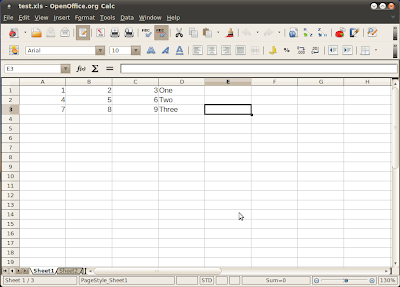This course will become read-only in the near future. Tell us at community.p2pu.org if that is a problem.

# Objective

In this session you will learn the following:

1. Reading data from Microsoft files

# Introduction

Scilab can read numbers and text stored in Microsoft Excel files. As of Scilab version 5.3.2, only Microsoft Excel 2003 or older files can be read. Microsoft 2007 and Microsoft 2010 files cannot be read (If you have data in the newer versions of Microsoft Excel, you can choose File ->Save as and choose an older version to be able to read from Scilab). Since OpenOffice.org Calc and a number of other Office applications can write Excel files, this discussion is applicable not just to Microsoft Windows and Microsoft Excel but a variety of operating systems and office spreadsheet applications.

Importing dat afrom a Microsoft Excel file into Scilab variables simplifies data input and makes it possible to apply more sophisticated processing than what can be done in Microsoft Excel.

# Functions Used

To demostrate the use of these functions, let us first create a Microsoft Excel file with the following data and save it Microsoft Excel 97-2003 format (.xls extension).The complete operation involves the following steps:

1. Open the Microsoft Excel file with the xls_open() function.
3. Close the file with the mclose() function.

The syntax of xls_open() is as follows:

[fd, sst, sh_names, sh_pos] = xls_open(filename)

Function xls_open() takes one input argument:

1. A string with the file name (including the full path if needed). It is a good idea to check the current working directory and change it to the directory where the file to be read is located before commencing with the read operation.

Function xls_open() requires the following output arguments:

1. fd - File descriptor of the file opened for reading. The file must exist, otherwise the function returns an error.
2. sst - A vector of all character strings in the Excel sheets
3. sh_names - A vector of strings containing the names of the sheets in the Excel file
4. sh_pos - A vector of numbers containing the beginning of different sheets within the Excel file

-->[fd, sst, sh_names, sh_pos] = xls_open("test.xls");
-->fd
fd  =
1
-->sst
sst  =
!One  Two  Three  !
-->sh_names
sh_names  =
!Sheet1  Sheet2  Shet3  !
-->sh_pos
sh_pos  =
11847   12441   12831
-->mclose(fd)

Match the output with the contents of the file and you will see that the string content of the Excel file and the sheet names match.

We can now read the data from the file, both numeric as well as text data.

text  =
0.    0.    0.    1.
0.    0.    0.    2.
0.    0.    0.    3.
numeric  =
1.    2.    3.   NaN
4.    5.    6.   NaN
7.    8.    9.   NaN
-->disp(text(1,4)) // Display text in cell (1,4) of sheet 1
One

This function takes two input arguments:

1. File descriptor to the the file obtained from the xls_open() function call to specify the file from which data is to be read.
2. Sheet position of the sheet from which data is to be read. To read data from the first sheet, use sh_pos(1), 1 being the number of the sheet to read the data from.

Function xls_read() returns two output values:

1. First matrix numeric contains all the numeric values in the sheet, with cells containing non-numeric data marked as NaN (Not a number)
2. Second matrix text contains index to the character data stored in the variable sst returned by xls_open(), with cells containing numeric data filled with zeros.

Function readxls() can accomplish the same task that we just completed using two functions xls_open() and xls_read(). It is simpler to use and more direct. Function readxls() takes one input argument, the name of the Excel file. It returns an mlist of type xls, containing one field named sheets. Data in an individual sheet can then be read from its fields, namely, value and text. Here is the procedure to read Excel files using readxls().

-->typeof(sheets)
ans  =
xls
-->s1 = sheets(1);
-->s1.value()
ans  =
1.    2.    3.   NaN
4.    5.    6.   NaN
7.    8.    9.   NaN
-->s1.text()
ans  =
!    One   !
!    Two   !
!    Three !

# Reading and Writing CSV Files

While xls_read() and readxls() functions enable Scilab to import data from Excel files, there are no files to export Scilab data to Excel format. However, Scilab has a function wite_csv() to export Scilab data as comma separated values (CSV) which can be imported into Excel. Thus Scilab data can be read into Excel indirectly. To test this, let us open the previous Excel file and save it as CSV file with the name test.csv.

Following example demonstrates how to read and write CSV files: